NCERT Solutions - Circle

# Circle NCERT Solutions - English for Class 1 (Marigold)

Page No 57:

Question 1: Learn New Words
A) Ball
B) Blue
C) Circle
D) Lines
E) Moon
A) Ball: A round-shaped object used for playing games Example sentences:
1) Ritika bought a new ball from the shop.
2) Cricket is played with a bat and a ball.

B) Blue: Colour of the sky Example sentences:
1) She was wearing a blue frock.
2) Our school building is blue in colour.

C) Circle: Round shape Example sentences:
1) Draw a circle in your notebook.
2) The teacher asked us to sit in a circle.

D) Lines: Long, unbroken marks Example sentences:
1) Draw a straight line.
2) Stand in a line.

E) Moon: A large round object that shines at night in the sky Example sentences:
1) I am not able to see the moon.
2) The moon is surrounded by many stars.

Page No 58:

Question 1:

• What did Grandmother and Mohini draw first?
• What were the colours of the balloons that Mohini drew?

• First, grandmother and Mohini drew circles.
• Mohini drew red, blue, green and yellow coloured balloons.

Question 2:

• In the circles below draw —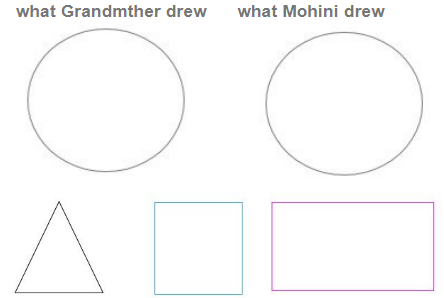what Grandmother drew      what Mohini drew Look at these shapes.
Try making these shapes into• a car • a house • a door • anything else
Disclaimer: Kindly refer the textbook for the image of the house.
Disclaimer:
Such activities are made for increasing self creative skills and should be tried by the students on their own.

Page No 59:

Question 1: Draw along the dots. So, what did you make? Can you make sounds like a train?
Draw a face on the balloon below. One has been done for you.
Disclaimer: Kindly refer the textbook for the images.
I made a train. Yes, I can make sounds like a train.
Disclaimer: Such activities are made for increasing self creative skills and should be tried by the students on their own.

Page No 60:

Question 1: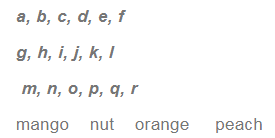Disclaimer: Kindly refer the textbook for the images.
Disclaimer:
Join the dotted lines to form the given alphabets and words.

Question 2: Look at the picture and fill in the blanks.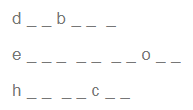Disclaimer: Kindly refer the textbook for the images.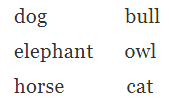The document Circle NCERT Solutions | English for Class 1 (Marigold) is a part of the Class 1 Course English for Class 1 (Marigold).
All you need of Class 1 at this link: Class 1

## English for Class 1 (Marigold)

28 videos|130 docs|23 tests

## FAQs on Circle NCERT Solutions - English for Class 1 (Marigold)

 1. What is a circle?Ans. A circle is a closed curved shape in which all points on the curve are equidistant from a fixed point called the center.
 2. What are the elements of a circle?Ans. The elements of a circle are the radius, diameter, circumference, and area. The radius is the distance from the center to any point on the circle. The diameter is a line segment that passes through the center and connects two points on the circle. The circumference is the distance around the circle, while the area is the region enclosed by the circle.
 3. How is the circumference of a circle calculated?Ans. The circumference of a circle can be calculated using the formula C = 2πr, where C represents the circumference and r represents the radius of the circle. Alternatively, the circumference can also be calculated using the formula C = πd, where d represents the diameter of the circle.
 4. How is the area of a circle calculated?Ans. The area of a circle can be calculated using the formula A = πr^2, where A represents the area and r represents the radius of the circle. This formula is derived by squaring the radius and multiplying it by the mathematical constant π (pi).
 5. How are circles used in real life applications?Ans. Circles have various real-life applications. They are used in designing wheels, gears, and pulleys, as well as in the construction of bridges and arches. Circles are also utilized in navigation systems, such as GPS, and are important in understanding concepts like orbits in astronomy and the movement of planets.

## English for Class 1 (Marigold)

28 videos|130 docs|23 tests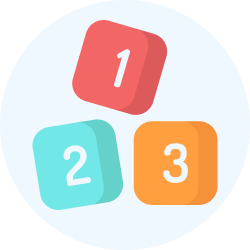Explore Courses for Class 1 examSignup to see your scores go up within 7 days! Learn & Practice with 1000+ FREE Notes, Videos & Tests.
10M+ students study on EduRev
Track your progress, build streaks, highlight & save important lessons and more!
Related Searches

,

,

,

,

,

,

,

,

,

,

,

,

,

,

,

,

,

,

,

,

,

;